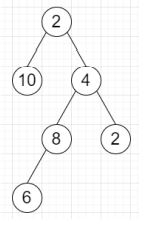# Program to find longest even value path of a binary tree in Python

PythonServer Side ProgrammingProgramming

Suppose we have a binary tree, we have to find the longest path consisting of even values between any two nodes in the tree.

So, if the input is likethen the output will be 5 as the longest path is [10, 2, 4, 8, 6].

To solve this, we will follow these steps −

• ans := 0

• Define a function find() . This will take node

• if node is null, then

• return (-1, -1)

• leftCnt := maximum of returned value of find(left of node) + 1

• rightCnt := maximum of returned value of find(right of node) + 1

• if value of node is even, then

• ans := maximum of ans and (leftCnt + rightCnt + 1)

• return (leftCnt, rightCnt)

• otherwise,

• ans := maximum of ans, leftCnt and rightCnt

• return (-1, -1)

• From the main method do the following −

• find(root)

• return ans

Let us see the following implementation to get better understanding −

## Example

class TreeNode:
def __init__(self, val, left=None, right=None):
self.val = val
self.left = left
self.right = right
class Solution:
def solve(self, root):
ans = 0
def find(node):
nonlocal ans
if not node:
return -1, -1
leftCnt = max(find(node.left)) + 1
rightCnt = max(find(node.right)) + 1
if node.val % 2 == 0:
ans = max(ans, leftCnt + rightCnt + 1)
return leftCnt, rightCnt
else:
ans = max(ans, max(leftCnt, rightCnt))
return -1, -1
find(root)
return ans
ob = Solution()
root = TreeNode(2)
root.left = TreeNode(10)
root.right = TreeNode(4)
root.right.left = TreeNode(8)
root.right.right = TreeNode(2)
root.right.left.left = TreeNode(6)
print(ob.solve(root))

## Input

root = TreeNode(2)
root.left = TreeNode(10)
root.right = TreeNode(4)
root.right.left = TreeNode(8)
root.right.right = TreeNode(2)
root.right.left.left = TreeNode(6)

## Output

5